### Observation of a new neutral baryon

In the origin (late 1960s) the particle zoo(1) is the colloquially word used to describe the extensive list of known elementary particles. Indeed, before Standard Model becames the more accepted theory in particle physics, physicists discovered a lot of particles in their accelerators, but we know today that they are simply a combination of a little numbers of particles classified in three fundamental families: leptons, quarks (that they constitute fermions, particles with half-integer spin) and bosons (particles with integer spin).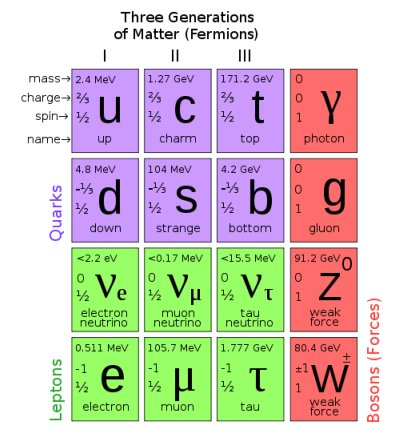(Commons)
We can classify also particles in a lot of sub-families, like baryons, the heavy particles constituted by three quarks: for example proton and neutron are barions, with the following composition: uud and udd respectively, where u is the up quark and d the down quark.
We know six types of quarks: up (u) and down (d), that explain protons and neutrons, charm (c), strange (s), top (t) and bottom (b) that explain a lot of other heavy particles. Standard Model predicts a series of combination of this quarks that they are summirized in a picture like this: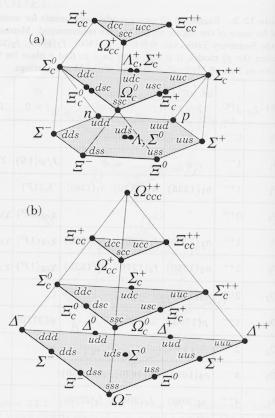(source)
In the up there is with angular momentum $J =1/2$ and down with angular momentum $J=3/2$. Today we examine $J=1/2$ group, in particular to the last discover by CDF, one collaboration at Tevatron in Fermilab. Indeed, not all particles predicted by SM are found, and the hunt to them is open. On the 20th July, Pat Lukens announced the first observation of $\Xi_b^0$, a baryon with the structure usb: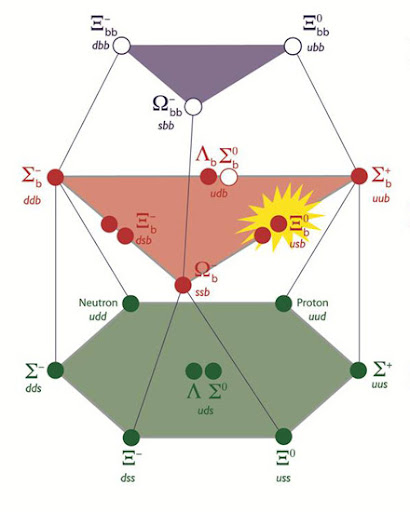In orther to detect the new baryon, researchers at Tevatron must reconstruct the following decay chain:
$\Xi_b^0 \rightarrow \Xi_c^+ \pi^-$
$\Xi_c^+ \rightarrow \Xi^- \pi^+ \pi^+$
$\Xi^- \rightarrow \Lambda \pi^-$
$\Lambda \rightarrow p \pi^-$
We can visualize the chain with the following diagram: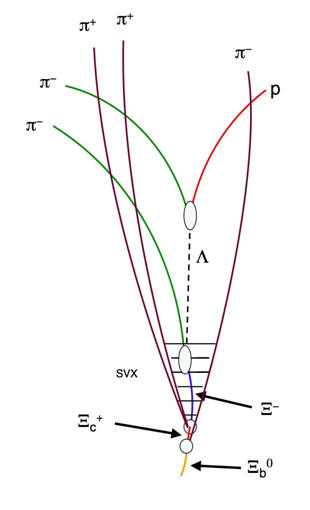So you can see that is very difficult determine the decay channel in which Fermilab is interested, indeed the great part of the preprint (via ScienceDaily) is devoted to describe the procedure used to select the right channel.
After this very long work, researchers found a peak in $\Xi_c^0 \pi^-$ (a) and $\Xi_c^+ \pi^-$ (b) distributions: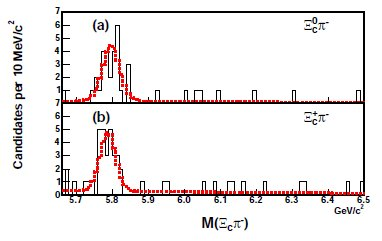and probably correspond to a particle with mass $m = 5787.8 \pm 5.0 (\text{stat}) \pm 1.3 (\text{syst}) MeV/c^2$.
But the preprint presents also an interesting analysis that I rarely read in paper of this kind, the reconstruction of the trajectories using the transverse flight distance $f(h)$ of hadron $h$, which is the distance travelled by a given particle in transverse view.
It can distingush between neutral and charged particles. In the first case the tfd is given by
$f(h) = \left (\vec{r_d} - \vec{r_o} \right ) \frac{\vec{p_T}(h)}{\left | \vec{p_T}(h) \right |}$
where $\vec{r_d}$ is the particle's decay position, $\vec{r_o}$ is a origin point, $\vec{p_T}$ the transverse momentum.
For charged particle $f(h)$ is calculated as the arc lenght in the transverse viem from $\vec{r_c}$ to $\vec{r_d}$, where $\vec{r_c}$ is the closet point to $\vec{r_o}$ in the particle's trajectory.
$f(h)$ is also used to calculate the proper decay time of weakly decaying states(2):
$t = f(h) \frac{M(h)}{c \left | \vec{p_T}(h) \right |}$
where $M(h)$ is the reconstructed mass of the particle.
Another tool used for the reconstruction of trajectories is the transverse impact distance $d(h)$, the distance between $\vec{r_c}$ and $\vec{r_o}$. For neutral particle is given by:
$d(h) = \frac{\left | \left ( \vec{r_d}-\vec{r_o} \right ) \times \vec{p_T}(h) \right |}{\left | \vec{p_T}(h) \right |}$
and for charged particle is simply
$d(h) = \left | \vec{r_c}-\vec{r_o} \right |$
The discover of $\Xi_b^0$, if confirmed, is added to the previous Fermilab's discovers of $\Xi_b^-$ (dsb), $\Sigma_b^*$ (uub, ddb), $\Omega_b$ (ssb).

Probably you know the site, but Particle Data Group is the particle's bible: if $\Xi_b^0$ will be confirmed, this is the place in which the baryon will be signed!
And finally I suggest you to watch a very funny video about particle zoo.
Enjoy!

P.S.: thanks to Marco Delmastro and Tommaso Dorigo for sharing the news!

(2) The weak interaction has vary short tipical times: for example W and Z bosons lived about $10^{-24}$ s, or $\pi^0$ lived for $10^{-16}$ s, but there is also neutron that lives for 15 minutes.